Question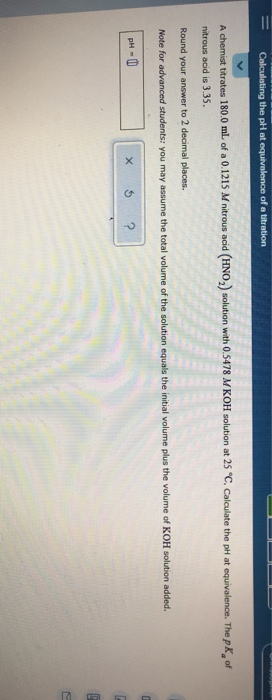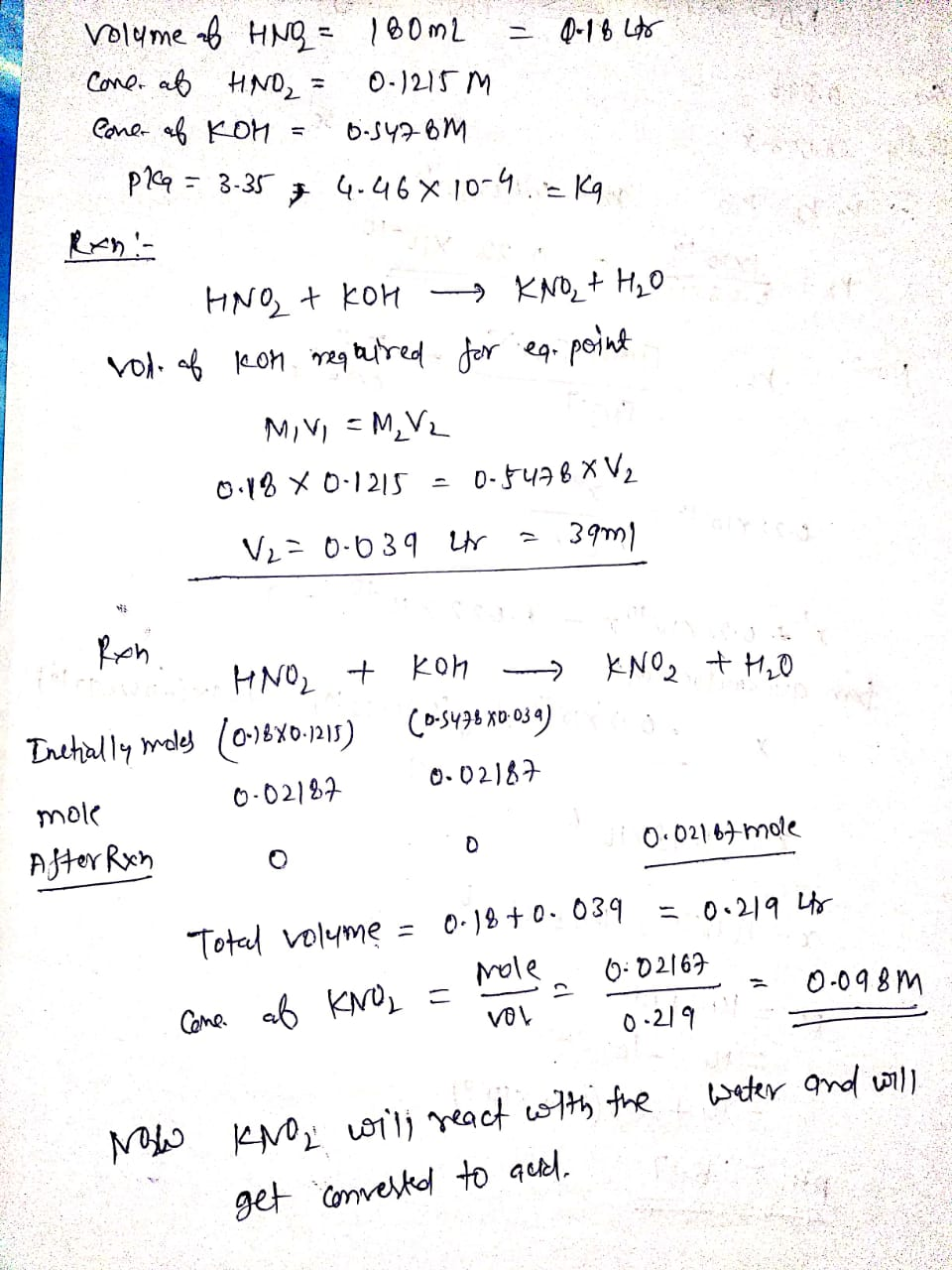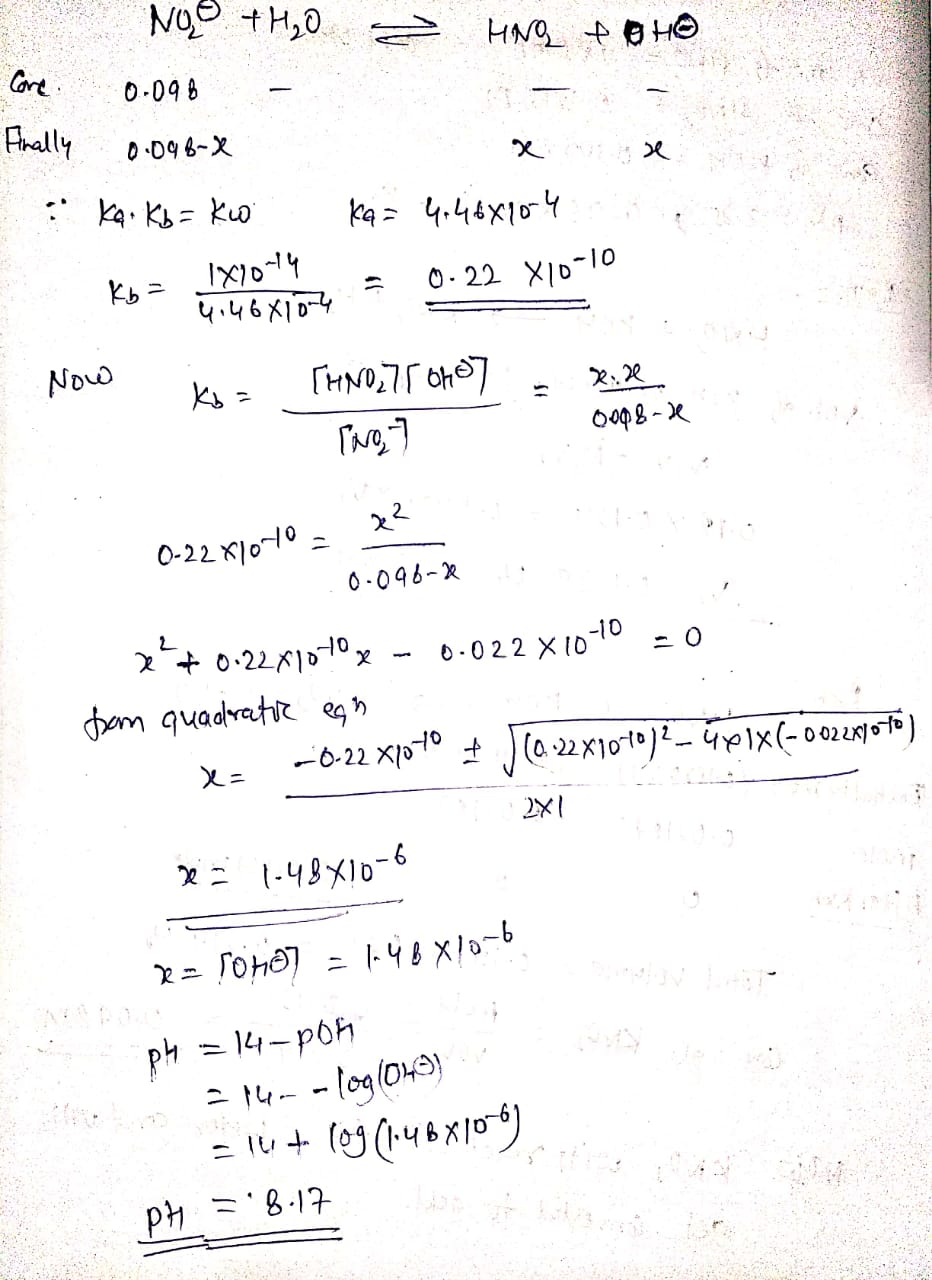#### Earn Coins

Coins can be redeemed for fabulous gifts.

Similar Homework Help Questions
• ### ACIDS AND BASES Calculating the pH at equivalence of a titration A chemist titrates 130.0 mL...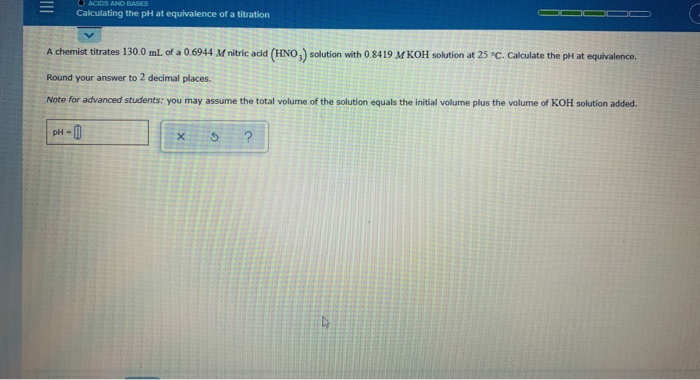ACIDS AND BASES Calculating the pH at equivalence of a titration A chemist titrates 130.0 mL of a 0.6944 M nitric acid (HNO.) solution with 0.8419 M KOH solution at 25 °C. Calculate the pH at equivalence Round your answer to 2 decimal places. Note for advanced students: you may assume the total volume of the solution equals the initial volume plus the volume of KOH solution added. pH-0 X 5 ?

• ### Calculating the pH at equivalence of a titration A chemist titrates 120.0 mL of a 0.7513...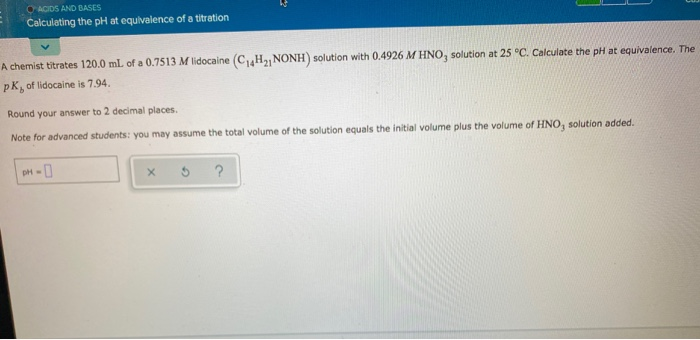Calculating the pH at equivalence of a titration A chemist titrates 120.0 mL of a 0.7513 M lidocaine (C4H2, NONH) solution with 0.4926 M HNO, solution at 25°C. Calculate the pH at equivalence. The PK, of lidocaine is 7.94 Round your answer to 2 decimal places Note for advanced students: you may assume the total volume of the solution equals the initial volume plus the volume of HNO, solution added. DH-

• ### = O ACIDS AND BASES Calculating the pH at equivalence of a titration A chemist titrates...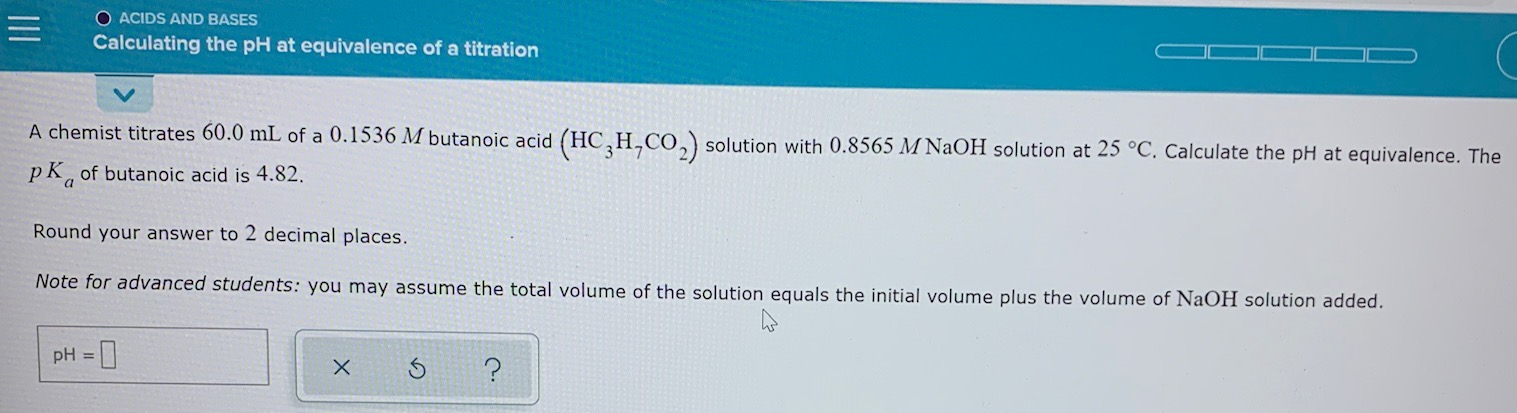= O ACIDS AND BASES Calculating the pH at equivalence of a titration A chemist titrates 60.0 mL of a 0.1536 M butanoic acid (HC,H,CO2) solution with 0.8565 M NaOH solution at 25 °C. Calculate the pH at equivalence. The pk, of butanoic acid is 4.82. Round your answer to 2 decimal places. Note for advanced students: you may assume the total volume of the solution equals the initial volume plus the volume of NaOH solution added. pH = x...

• ### ACIDS AND BASES Calculating the pH at equivalence of a titration A chemist titrates 200.0 mL...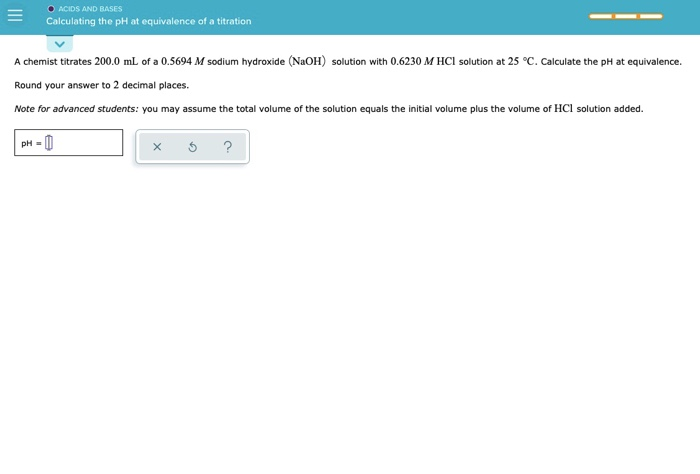ACIDS AND BASES Calculating the pH at equivalence of a titration A chemist titrates 200.0 mL of a 0.5694 M sodium hydroxide (NaOH) solution with 0.6230 M HCl solution at 25 °C. Calculate the pH at equivalence. Round your answer to 2 decimal places. Note for advanced students: you may assume the total volume of the solution equals the initial volume plus the volume of HCl solution added. PH-0 5 ?

• ### calculating the ph of a weak acid titrated with a strong base An analytical chemist is...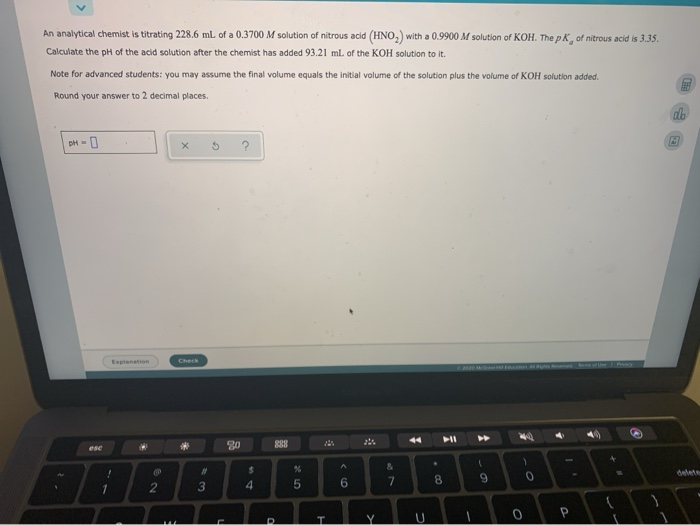calculating the ph of a weak acid titrated with a strong base An analytical chemist is titrating 228.6 ml. of a 0.3700 M solution of nitrous acid (HNO,) with a 0.9900 M solution of KOH. The pk of nitrous acid is 3.35. Calculate the pH of the acid solution after the chemist has added 93.21 ml of the KOH solution to it. Note for advanced students: you may assume the final volume equals the initial volume of the solution plus...

• ### Calculating the ph of a weak acid bitrated with a strong base An analytical Chemist is...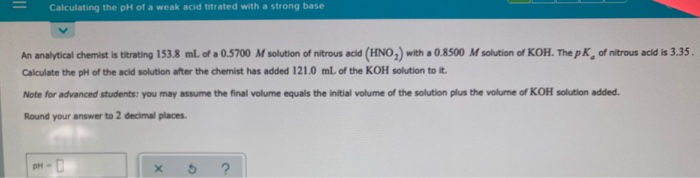Calculating the ph of a weak acid bitrated with a strong base An analytical Chemist is titrating 153.8 ml. of a 0.5700 M solution of nitrous acid (HNO,) with a 0.8500 M solution of KOH. The pk of nitrous acid is 3.35. Calculate the pH of the acid solution after the chemist has added 121.0 ml. of the KOH solution to it. Note for advanced students: you may assume the final volume equals the initial volume of the solution plus...

• ### - Calculating the pH of a weak acid titrated with a strong base An analytical chemist is titrating 123.0 ml. of a 0...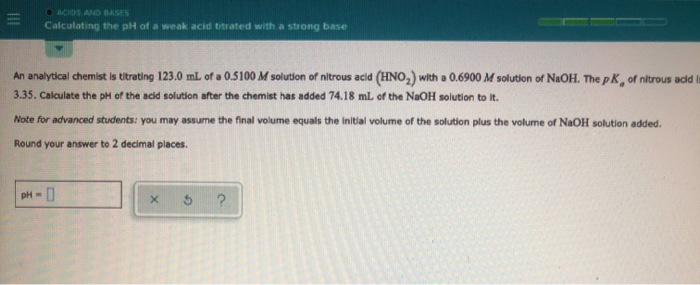- Calculating the pH of a weak acid titrated with a strong base An analytical chemist is titrating 123.0 ml. of a 0.5100 M solution of nitrous acid (HNO,) with a 0.6900 M solution of NaOH. The pk of nitrous add 3.35. Calculate the pH of the acid solution after the chemist has added 74.18 ml of the NaOH solution to it. Note for advanced students you may assume the final volume equals the initial volume of the solution plus...

• ### Calculating the pH at equi.. A chemist titrates 70.0 ml. of a 04557 M carbonic acid...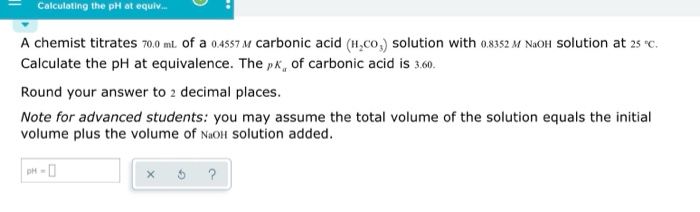Calculating the pH at equi.. A chemist titrates 70.0 ml. of a 04557 M carbonic acid (H,co,) solution with o8352 M NaOlH Solution at 2s c Calculate the pH at equivalence. The pk, of carbonic acid is 3.60 Round your answer to 2 decimal places. Note for advanced students: you may assume the total volume of the solution equals the initial volume plus the volume of NaoH Solution added. рH - D

• ### An analytical chemist is titrating 118.8 mL of a 0.2500 M solution of nitrous acid (HNO)...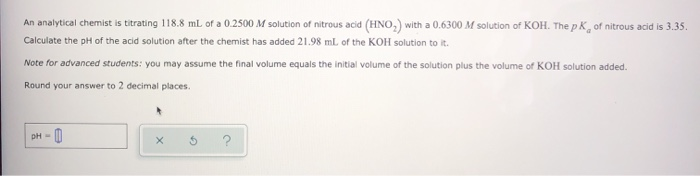An analytical chemist is titrating 118.8 mL of a 0.2500 M solution of nitrous acid (HNO) with a 0.6300 M solution of KOH. The pk of nitrous acid is 3.35. Calculate the pH of the acid solution after the chemist has added 21.98 ml of the KOH solution to it. Note for advanced students: you may assume the final volume equals the initial volume of the solution plus the volume of KOH solution added. Round your answer to 2 decimal...

• ### Calculating the ph of a weak acid bitrated with a strong base An analytical Chemist is...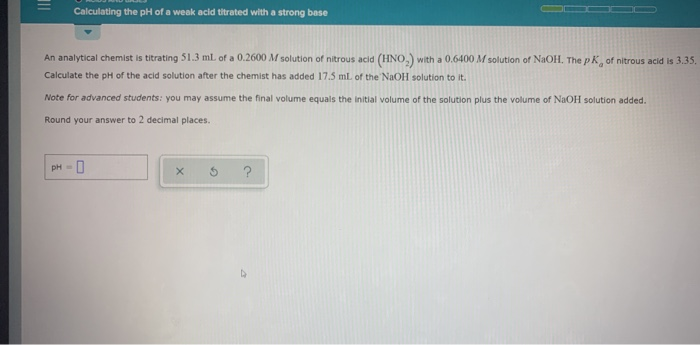Calculating the ph of a weak acid bitrated with a strong base An analytical Chemist is titrating 51.3 ml of a 0.2600 M solution of nitrous acid (HNO.) with a 0.6400 M solution of NaOH. The pk of nitrous acid is 3.35 Calculate the pH of the acid solution after the chemist has added 17.5 mL of the NaOH solution to it. Note for advanced students: you may assume the final volume equals the initial volume of the solution plus...